# WORKING WITH TUPLE IN PYTHON

in STEMGeeks5 months ago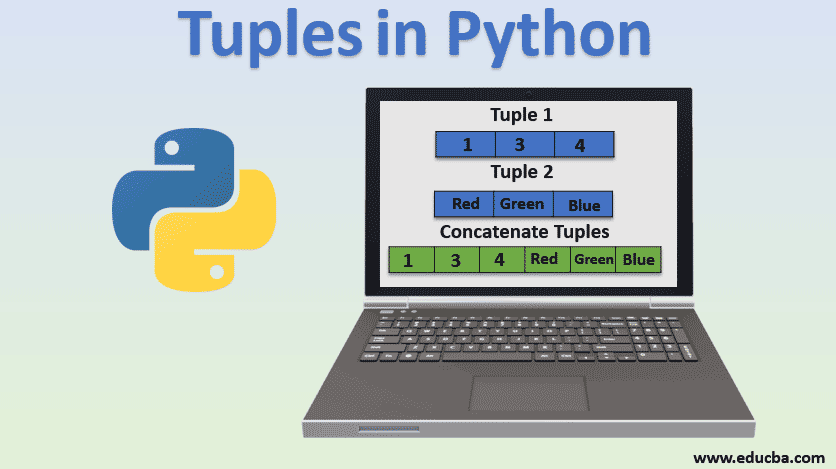Image Source

Hey guys, after my tutorial on Python List, I want to show you another important data types called Tuple. You can find my tutorial on list with practical examples here. The same post contains the link to the first part of the tutorial with list too.

Tuple works like a list in python although there is some differences between them. List can be used to store value in a particular sequence and is used only if you want to modify the value i.e. update the value or delete the value. However tuples are immutable data types i.e. once it is created, it can't be modified. List is a mutable data types meaning it can be changed even after it is created. Other mutable data types in python include set, dictionary etc. Other examples of immutable data types include integer, boolean, float etc. So we use tuples only if we don't want to modify the value. Other difference is that to define tuple, we used parentheses (round brackets) whereas for list you use square brackets.

Now lets see some simple examples on tuple. Open your desired IDE and create a new file. Named it as anything you like. Lets create a simple tuple that holds only numerical value and then we will print it.

``````number = (34, 22, 76, 90, 43, 9, 51, 88, 13)
print(number)
``````

This will print a tuple for us as below: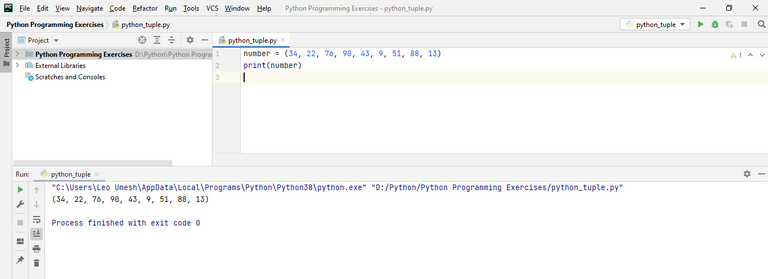Accessing the element of tuple is same as of list. We just specify the index number by writing tuple name followed by square bracket inside which index number is written. Also, note that index number is 0 for the first element in the tuple and the series go on. So if we want to access value which is 90 in the above code, we should specify index number as 3.

``````number = (34, 22, 76, 90, 43, 9, 51, 88, 13)
print(number)
``````

This code will print our desired output: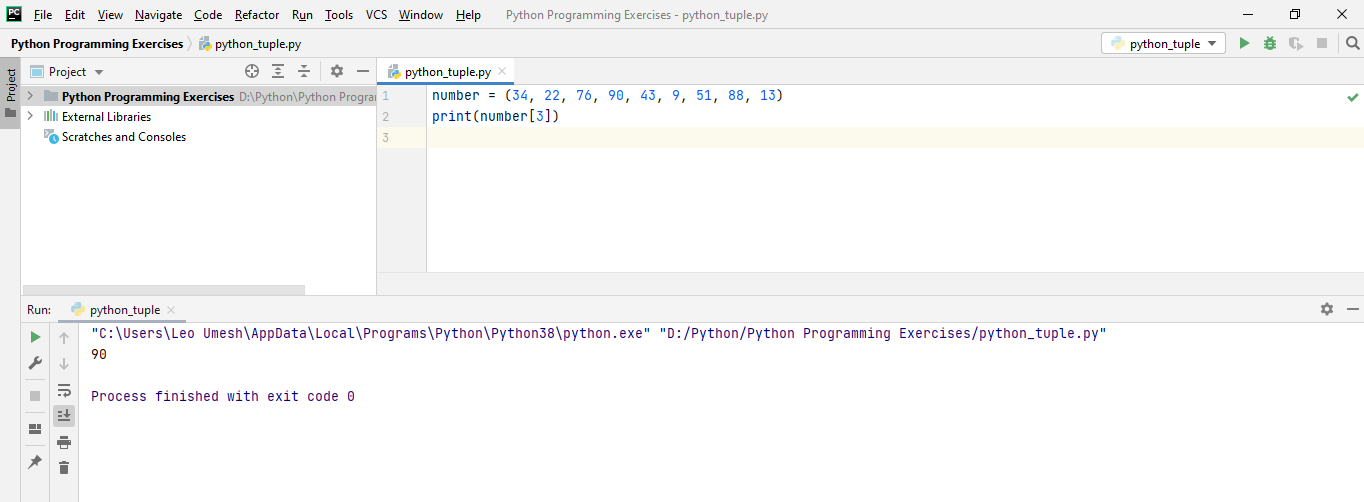Now let me show you why tuples are immutable. Lets suppose we want to change this value from 90 to 92. Then as we do in list, we will do the same with tuple and lets print the modified list.

``````number = (34, 22, 76, 90, 43, 9, 51, 88, 13)
number = 92
print(number)
``````

You can see in below image when I executed this code. PyCharm already highlighted second line even before I run this code. And it throws an error as 'tuple' object does not support item assignment which means you can't change the value of items in the tuple.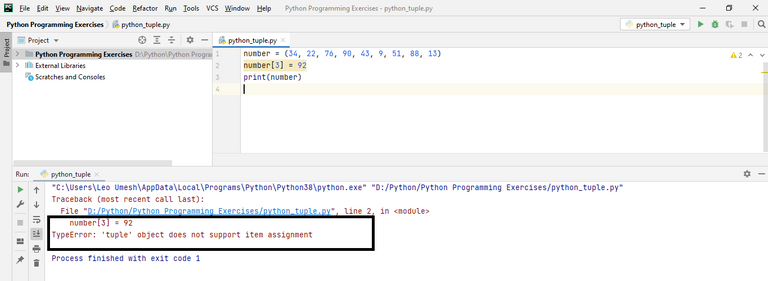You can also slice a tuple as we did in the list using exact same syntax. I won't be doing that here. You can see the tutorials on list above and try to do the same with tuples. Just for a quick demo here, I will show you the simplest slice. I want to print everything from the starting to the end skipping each element in between. You have to add one to the index at last if you want to print the last element too.

``````number = (34, 22, 76, 90, 43, 9, 51, 88, 13)

print(number[0:9:2])
``````

The output will be as below. 0 means the starting point, 9 means the ending point (it will print up to index number 8 which we desire) and 2 means to skip one value in between. Write 3 if you want to skip 2 values in between.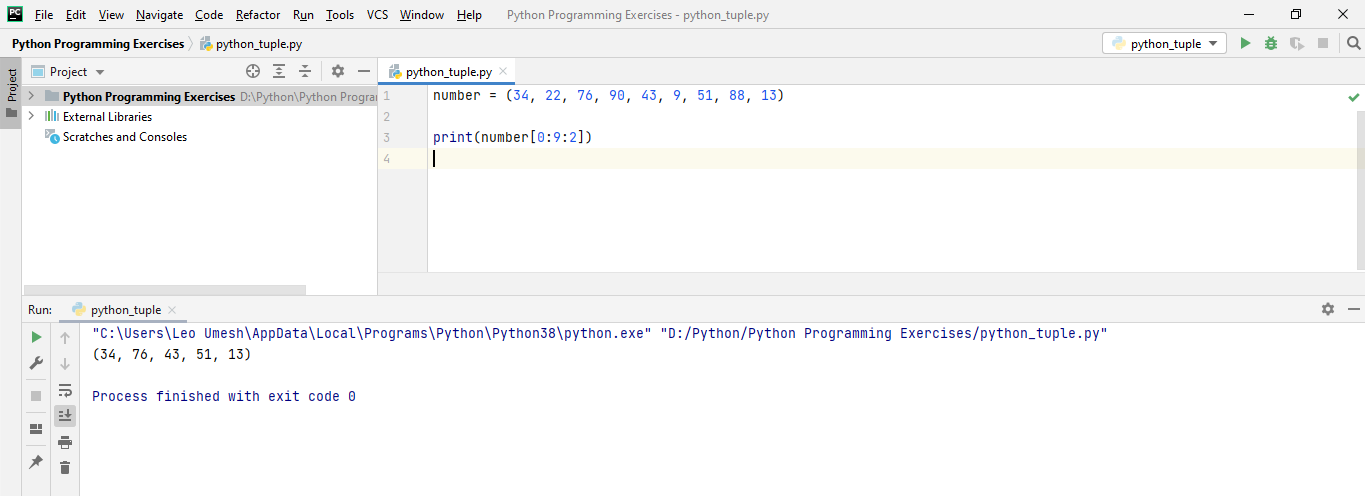There's one indirect way to make tuple mutable. You can first convert the tuple into the list and then modify its value and again convert that list to tuple. I have this code that will summarize this all:

``````number = (34, 22, 76, 90, 43, 9, 51, 88, 13)

number_list = list(number)

print(number_list)

number_list = 92

print(number_list)

number = tuple(number_list)

print(number)
``````

First we have a tuple called as `number`. Then we used a method called `list()` that converts our `number` tuple to a list and we stored this in a variable called `number_list`. We modified the fourth element (index 3) value from 90 to 92 of `number_list`. Then at last we used a method called `tuple()` that converts our `number_list` to a tuple and we stored this tuple in our original variable called `number`. In each step, I am using `print()` statement to show what's actually is being happened behind the scene.

The output of this code upon execution is as below: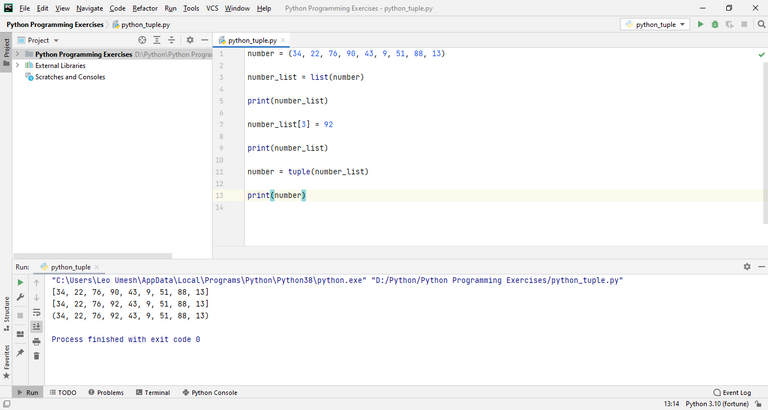Let me know if you have any doubts, concerns or feedbacks for me below🙂.